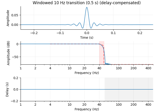# mne.viz.plot_ideal_filter#

mne.viz.plot_ideal_filter(freq, gain, axes=None, title='', flim=None, fscale='log', alim=(-80, 10), color='r', alpha=0.5, linestyle='--', show=True)[source]#

Plot an ideal filter response.

Parameters:
freqarray-like

The ideal response frequencies to plot (must be in ascending order).

gain

The ideal response gains to plot.

axesinstance of `Axes` | `None`

The subplot handle. With None (default), axes are created.

title`str`

The title to use, (default: ‘’).

flim

If not None, the x-axis frequency limits (Hz) to use. If None (default), freq used.

fscale`str`

Frequency scaling to use, can be “log” (default) or “linear”.

alim`tuple`

If not None (default), the y-axis limits (dB) to use.

colorcolor object

The color to use (default: ‘r’).

alpha`float`

The alpha to use (default: 0.5).

linestyle`str`

The line style to use (default: ‘–‘).

showbool

Show figure if True (default).

Returns:
figinstance of `matplotlib.figure.Figure`

The figure.

Notes

New in version 0.14.

Examples

Plot a simple ideal band-pass filter:

```>>> from mne.viz import plot_ideal_filter
>>> freq = [0, 1, 40, 50]
>>> gain = [0, 1, 1, 0]
>>> plot_ideal_filter(freq, gain, flim=(0.1, 100))
<...Figure...>
```

## Examples using `mne.viz.plot_ideal_filter`#Background information on filtering

Background information on filtering